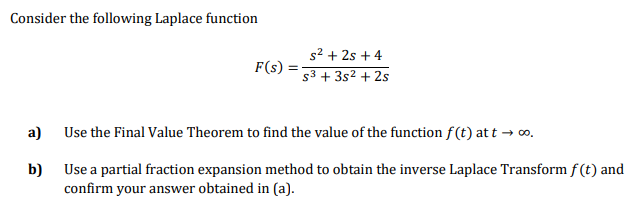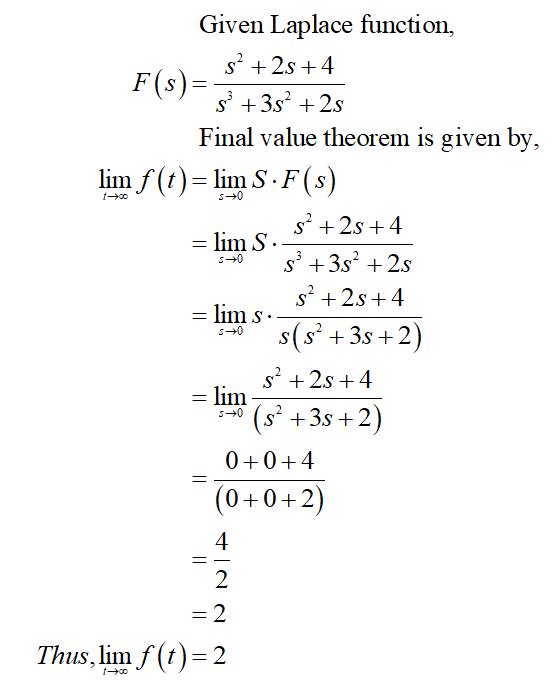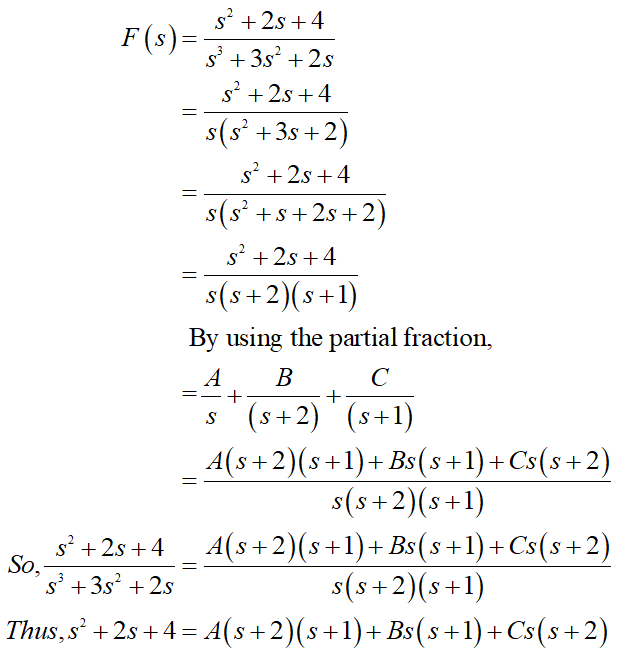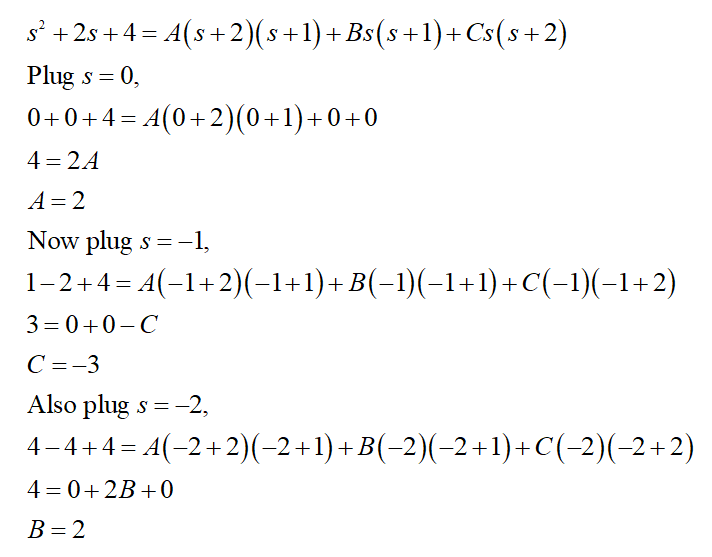# Consider the following Laplace functions2 + 2s + 4s3 + 3s2 + 2sF(s)Use the Final Value Theorem to find the value of the function f(t) at t → o.a)Use a partial fraction expansion method to obtain the inverse Laplace Transform f (t) andb)confirm your answer obtained in (a).

Question
24 viewshelp_outlineImage TranscriptioncloseConsider the following Laplace function s2 + 2s + 4 s3 + 3s2 + 2s F(s) Use the Final Value Theorem to find the value of the function f(t) at t → o. a) Use a partial fraction expansion method to obtain the inverse Laplace Transform f (t) and b) confirm your answer obtained in (a). fullscreen
check_circle

Step 1

Consider the given question,

(a)Step 2

(b)

Inverse Laplace Transform f(t) is find as,Step 3

Now, substitute the value of s = 0, -1, -2 in the above obtained equation,...

### Want to see the full answer?

See Solution

#### Want to see this answer and more?

Solutions are written by subject experts who are available 24/7. Questions are typically answered within 1 hour.*

See Solution
*Response times may vary by subject and question.
Tagged in